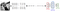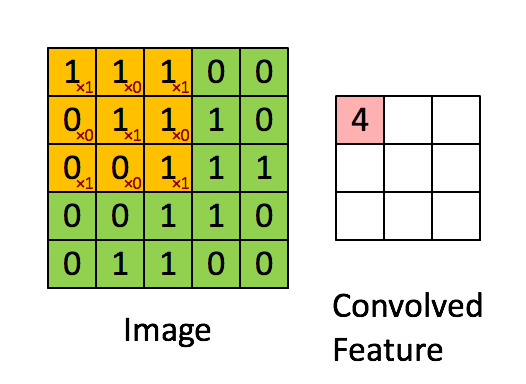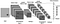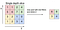# The Deep Learning Era — Part II

In the last post we saw that how can we reduce our dependency on domain expertise for NLP problems such as question answering by moving from a plethora of NLP statistical techniques to an end-to-end deep neural language based model. We also introduced a deep learning architecture using word embeddings and LSTM components without giving much insights into it and why we chose that architecture.

In fact, that architecture was inspired from one of those “easy to understand” research paper and implemented at my workplace by me using the Keras framework.

In this post we take a look at two of the most commonly used (apart from word2vec or word embeddings) layers of a deep neural network i.e. CNN and RNN (LSTM). There are so many cool blog posts and tutorials on both of them that I find it redundant to explain the fundamentals again.

Given a set of images belonging to fixed set of categories (e.g. cats vs. dogs, men vs. women etc.), what approach should we take to train a model to correctly classify an unknown image into one of those categories. Traditional methods would first grayscale the images (and most likely add some image processing on top of it like rotate, crop, blur etc.) and finally flatten out the pixels (pixels in a grayscale image are arranged into a 2D grid) into a 1D array, and then use the pixel values as inputs to a SVM or a neural net classifier. (A colored image would have a 3rd dimension for the RGB values).Grayscale image -> 2D Grid of Pixels -> Flatten out into 1D array -> Neural Network with pixel values from 1D array as inputs.

But notice that once we flatten a 2D image into a 1D array of pixels, we lose information at the edges as well as information about neighboring pixels. But then how do we train a classifier such as a SVM or a neural network which only works with inputs of shape of a 1D array. How about we encode the information about neighboring pixels and pixels at edges into a single value and repeat this successively until finally we have arrived at an encoded matrix which can be flattened “safely” out to a 1D array and trained with a SVM or neural net. Basically we are using 2D hidden layers here.The big green matrix is reduced to the smaller pink matrix by convolving the green matrix with the smaller yellow matrix.

The yellow matrix [[1, 0, 1], [0, 1, 0], [1, 0, 1]] is an encoding function, that slides over the green matrix and produces a single encoded value from 9 neighboring pixel values each time, in that way even if we flatten out the smaller pink matrix, we would have still retained some information about the neighborhood pixel values.

Big matrix of pixels →(Magic happens) → Smaller matrix (encoded) →(Again magic happens) →Still smaller matrix(encoded) →…and so on, →Smallest possible matrix that can be safely flattened without the fear of losing neighboring pixel information.Multiple layers of encoding of neighborhood pixels until flattening out before training classifier. (from mdpi.com)

The part where the “magic happens” is where CNN comes into picture. Each layer of CNN applies two stage hidden layer. In the first layer, we have these small matrices (known as filters or feature maps) that slide and convolve over the entire bigger matrix and produces a smaller encoded matrix. One feature map produces one smaller encoded matrix from one larger matrix. There can be multiple such f.maps and hence the output is an array of 2D matrices, each produced by one filter.

In the second stage, we have something called the pooling layer, that further reduces the size of the encoded matrix by 1/2 or 1/3 by pooling the maximum value from neighboring values in the encoded matrix.Max pooling. The 4x4 matrix is reduced to a 2x2 matrix by taking the maximum value out of each of the 4, 2x2 sub-matrices in the bigger matrix. (from cs231n course material)

We can have either one such layer of CNN or multiple such layers stacked one above the other. With multiple layers of CNN stacked on top of one another each layer learns composition of features (i.e. more complex features) from the layer below it. For example, if a certain layer learns to detect edges, corners and arcs in an image, then the layer above it might be able to detect shapes and objects.

How can CNN work with text data given that text data is inherently 1D ? Remember that, word2vec produces a vector for each word in a sentence, and thus a sentence can be represented as a 2D matrix (array of word vectors). But unlike in image where one pixel value in the 2D matrix represents one unit, in a text sentence, each word or more precisely each word vector is a unit. With image, filters can slide along both the X and Y axis, whereas with text, each filter (of length equal to the dimension of the word vector) can only move like a sliding window over the words but not along the word vector dimension.

Each word above is represented by a 5-length vector. 6 different feature maps are applied, 2 of size 4 (i.e. covering 4 words at a time), 2 of size 3 (covering 3 words at a time) and 2 of size 2. When we slide a size 4 map over 7 words, we get 4 scalar values and similarly sliding a size 3 map over the 7 words returns 5 scalar values and so on. Then after pooling, concatenate these scalars into a resultant vector and train a binary classifier.

But one can ask the question that why do we need CNN for text classification given there are already effective and popular algorithms such as XGBOOST, Neural Networks, Random Forests or SVM ? All the above algorithms works with TF-IDF scalar values of bag-of-words or N-Grams. If you analyze, then the above approach with CNN is same as the N-Gram approach, only difference is that the sliding window is over word vectors instead of words and word vectors can capture “meanings” of words.

There has been lots of work on using CNN for text classification as well as learning word and phrase embeddings instead of a Skip-Gram model.

What are some current applications of convolutional neural networks other than image, video and speech analysis?

Written by